# Escape-Time Fractals

 Burning Ship Julia Lyapunov Mandelbrot Newton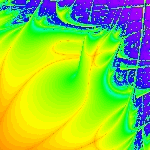The new Fractals package makes it easier to create and explore popular fractals, including the Mandelbrot, Julia, Newton, and other time-iterative fractals. The Fractals package can quickly apply various escape time iterative maps over rectangular regions in the complex plane, the results of which consist of images that approximate well-known fractal sets. You can further manipulate the fractal images using the ImageTools package, explore them interactively with the Exploration Assistant, and examine them with other computational commands.

The Fractals package also automatically applies multithreading and optimization techniques that take advantage of the full processing power of your computer by using the Compiler and evalhf for performance. This is further enhanced through use of the Threads package to achieve computational parallelism on multi-core platforms.

Color or grayscale images illustrate the underlying quasi-self-similarity of the fractal sets by exhibiting similar patterns at varying scales. By varying the domain, tolerance, and maximal iteration limit, a large variety of impressive images of fractals can be produced at user-defined resolutions.

 > with(Fractals:-EscapeTime):

Each of the four computational commands below generates three 400x400 pixel images. The first two grayscale images are Arrays, which store information about how many iterations of the relevant iterative map it takes from each starting point for the absolute value to exceed a tolerance (e.g. "escape") and what its final absolute value may be. Each color image is produced by combining the two grayscale layer Arrays to compute an Array with three color layers.

The CodeTools:-Usage command is called around the computations below in order to show the overall timings. The timings may vary, especially with repeated execution, according to whether garbage collection gets performed during the computation. The following commands were executed using 64-bit Maple 18 for Linux on a machine with an Intel(R) Core(TM) i5 CPU 760 @ 2.80GHz.

 > N := 400: outm := CodeTools:-Usage( Mandelbrot(N,-2-1.4*I,1+1.4*I,                                     output=[layer1,layer2,color]) );

 memory used=36.80MiB, alloc change=36.65MiB, cpu time=130.00ms, real time=95.00ms, gc time=0ns> outj := CodeTools:-Usage( Julia(N,-1.4-1.4*I,1.4+1.4*I, 0.37+0.37*I,                                output=[layer1,layer2,color]) ):

 memory used=36.80MiB, alloc change=36.65MiB, cpu time=150.00ms, real time=92.00ms, gc time=0ns

 > outb := CodeTools:-Usage( BurningShip(N,-1.8-0.09*I,-1.7+0.02*I,                                      output=[layer1,layer2,color]) ):

 memory used=36.81MiB, alloc change=-64.24MiB, cpu time=220.00ms, real time=149.00ms, gc time=50.00ms

 > outn := CodeTools:-Usage( Newton(N, -3.0-3.0*I, 3.0+3.0*I, z^3-2*z+2,                          output=[layer1,layer2,color]) ):

 memory used=30.64MiB, alloc change=30.55MiB, cpu time=980.00ms, real time=477.00ms, gc time=0ns

The resulting sequences of images can be embedded directly into the current worksheet, in a tabular format.

 > ImageTools:-Embed([[ outm ],[ outj ],[ outb ], [outn] ]);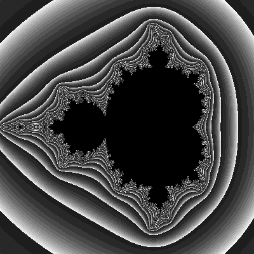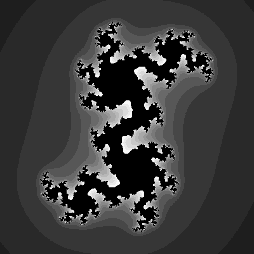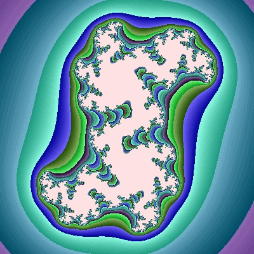The commands, which generate the fractal images, can also be dynamically investigated using the Explore command.

The following example shows an animated display of the colorized escape-time data demonstrating the Julia set.

 > Explore( Julia(300, -1.3-1.3*I, 1.3+1.3*I, 0.356-b*I, output=color)         , parameters=[ b=-0.75..0.75 ]         , animate, numframes=25         , placement=left );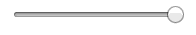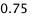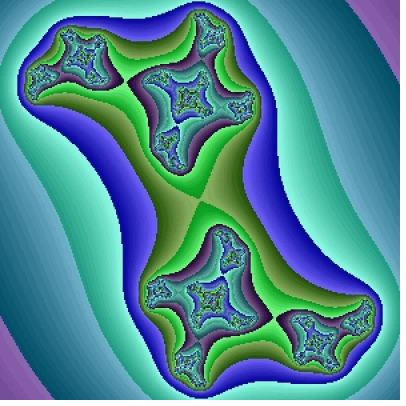## Bereit für den nächsten Schritt?

*Die Maple-Evaluation ist für Schüler und Studenten bzw. die private Nutzung zurzeit nicht verfügbar.